# Equation - math word problems

#### Number of problems found: 1347

• FlourKim needs 3/4 cup of flour to make 12 cookies. How much flour would she need to make 60 cookies?
• Sales of productsFor 80 pieces of two quality products a total sales is 175 Eur. If the first quality product was sold for n EUR per piece (n natural number) and the second quality product after 2 EUR per piece. How many pieces of the first quality were sold?
• Two shipsThe distance from A to B is 300km. At 7 am started from A to B a ferry with speed higher by 20 km/h than a ship that leaves at 8 o'clock from B to A. Both met at 10h 24min. Determine how far they will meet from A and when they reach the destination.
• Competition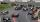The organizers of competion wanted to spent big amount of money to pay for the competitors. One third of this amount spent on prizes and the remaining € 5,000 to diplomas. How much was committed for rewards?
• SchoolsThree schools are attended by 678 pupils. To the first attend 21 students more and to the third 108 fewer students than to second school. How many students attend the schools?
• Bricks II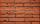3/4 bricks weighs 6 kg and 2/3 bricks. How weighs one whole brick?
• Two sisters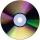Two sisters together have 54 CDs. 6/8 CDs has younger sister and is equal to 3/5 of CDs older sisters. How many CDs has each of the sisters?
• Summands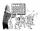Find two summands of the number 42, so that its product is minimized.
• Cuboid and eq2Calculate the volume of cuboid with square base and height 6 cm if the surface area is 48 cm2.
• Even / odd numbers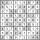a / Using variable n write two consecutive odd numbers. b / the sum of three consecutive odd numbers is 333. What are this numbers?
• AngleDetermine the size of the smallest internal angle of a right triangle which angles forming the successive members of the arithmetic sequence.
• Mushrooms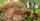Fresh mushrooms contain 90% water, dried only 12%. How many kilograms of fresh mushrooms are needed for 5kg dried?
• Apples 3Julka has 5 apples more than Hugo and four apples less than Annie. Hugo has 17 apples. How many apples has Julka and how Annie?
• Odd numbersThe sum of four consecutive odd numbers is 1048. Find those numbers ...
• Four numbersThe first number is 50% second, the second number is 40% third, the third number is 20% of the fourth. The sum is 396. What are the numbers?
• Vinegar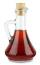What percentage vinegar we get if we mix 1 if dm³ eight percent vinegar with 1.5 dm³ six percent vinegar?
• The poolThe pool has a volume of 40 m3 and the water temperature is 20 °C. How much water at 100 °C should we pour into the pool to increase the water temperature by 5 °C?
• One percent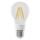One percent of all the lights in the city are LED, the remaining 99% are conventional. Other types are not there. John counted them honestly but he had counted only conventional. After a good dinner he registered numbers and he have notice that from all t
• Nice prismCalculate the surface of the cuboid if the sum of its edges is a + b + c = 19 cm and the body diagonal size u = 13 cm.
• Rectangle vs squareOne side of the rectangle is 1 cm shorter than the side of the square, the second side is 3 cm longer than the side of the square. Square and rectangle have the same content. Calculate the length of the sides of a square and a rectangle.

Do you have an interesting mathematical word problem that you can't solve it? Submit a math problem, and we can try to solve it.

We will send a solution to your e-mail address. Solved examples are also published here. Please enter the e-mail correctly and check whether you don't have a full mailbox.

Please do not submit problems from current active competitions such as Mathematical Olympiad, correspondence seminars etc...

Do you have a linear equation or system of equations and looking for its solution? Or do you have quadratic equation?